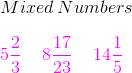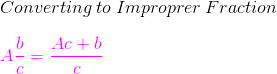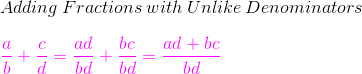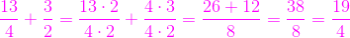# How to Add Mixed Fractions with Different Denominators

Instructor: Laura Pennington

Laura received her Master's degree in Pure Mathematics from Michigan State University. She has 15 years of experience teaching collegiate mathematics at various institutions, such as Grand Rapids Community College, Pikes Peak Community College, and Austin Peay State University.

In this lesson, we will take a close look at each of the steps involved in adding mixed fractions with different denominators. After familiarizing ourselves with the process, we will look at an application to put the process to use.

## Steps to Solve

To add mixed fractions with different denominators, we simply need to perform three steps. Of course, this means knowing how to do each step, so let's figure this out together. First of all, a mixed number is a number with a fraction part and a whole part.When we want to add mixed numbers with different denominators, the first step we take is to convert the mixed numbers to improper fractions, where an improper fraction is a fraction with a numerator larger than its denominator. When converting a mixed number to an improper fraction, the denominator will remain the same. To find the numerator, we multiply the whole part of the number by the denominator of the fraction part and then add the numerator of the fraction part.For example, suppose we are converting 3 1/4 to an improper fraction. We know the fraction's denominator remains the same, so it will be 4. To find the numerator, we multiply the whole number 3 by the denominator 4, and then add the numerator 1 to get 3*4 + 1 = 13. Thus, we have that 3 1/4 = 13 / 4.

The second step in adding mixed fractions with different denominators is to add together the two improper fractions that you found in the first step. To do this, you will need to find a common denominator and then add the numerators. This can be accomplished using the illustrated rule.For example, suppose we are adding 3 1/4 + 1 1/2. We already converted 3 1/4 to 13/4. To convert 1 1/2, we keep the denominator of 2 and find the numerator using our conversion rule. 1 1/2 = (1*2 + 1) / 2 = 3/2. Now, we add the improper fractions 13/4 and 3/2 using our addition rule.We see that 13/4 + 3/2 = 19/4.

The last step in adding mixed fractions with different denominators is to convert the improper fraction you found in the second step back to a mixed number. To do this, you perform the division indicated (in this case, 19 divided by 4). The quotient will be the whole number in the mixed number, the remainder will be the numerator in the fraction of the mixed number, and the denominator of the fraction in the mixed number stays the same.Consider our result 19/4 from our example. To convert this to a mixed number, we perform division to get 19/4 = 4 remainder 3. We now know that the whole number in the mixed number will be 4, the numerator of the fraction in the mixed number will be 3, and the denominator of the fraction in the mixed number will be 4. Therefore, 19/4 = 4 3/4.

Once you get familiar with these steps, adding mixed numbers with different denominators becomes a breeze! Let's look at these steps in a nice, laid out manner.

## Solution

1. Convert the mixed numbers to improper fractions.
2. Add the improper fractions together.
3. Convert the result back to a mixed number.

That's not too hard, is it?

To unlock this lesson you must be a Study.com Member.

### Register to view this lesson

Are you a student or a teacher?

#### See for yourself why 30 million people use Study.com

##### Become a Study.com member and start learning now.
Back
What teachers are saying about Study.com

### Earning College Credit

Did you know… We have over 200 college courses that prepare you to earn credit by exam that is accepted by over 1,500 colleges and universities. You can test out of the first two years of college and save thousands off your degree. Anyone can earn credit-by-exam regardless of age or education level.